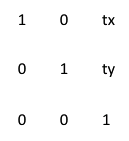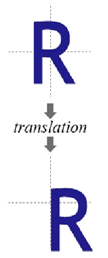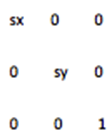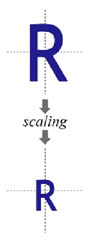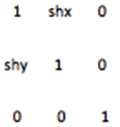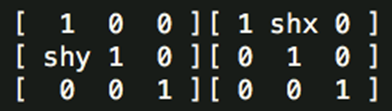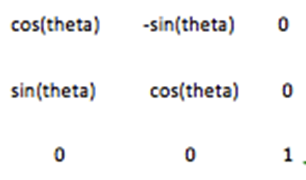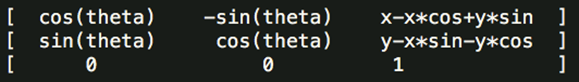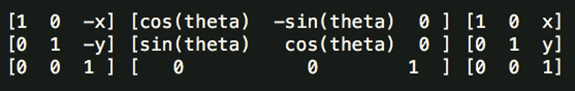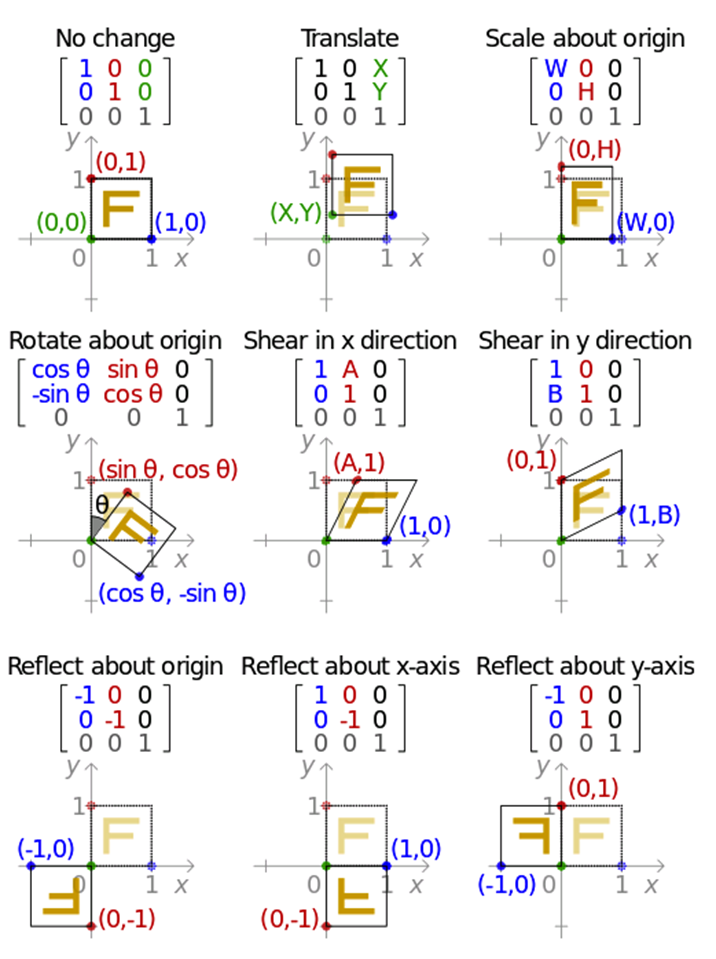# 图像处理刚体变换

## 数字图像处理：图像变换的基本模型

2019-10-04 19:01:53 Vichael_Chan 阅读数 375
• ###### 基于计算机视觉的无人驾驶感知系统

AI技术落地，给你的履历增加一个厉害的实训项目！ 掌握无人驾驶技术开发的重要知识点，进行实战。

8637课时 0分钟 343人学习 王而川
免费试看

## 数字图像处理：图像变换的基本模型

### 一、常用图象的变换模型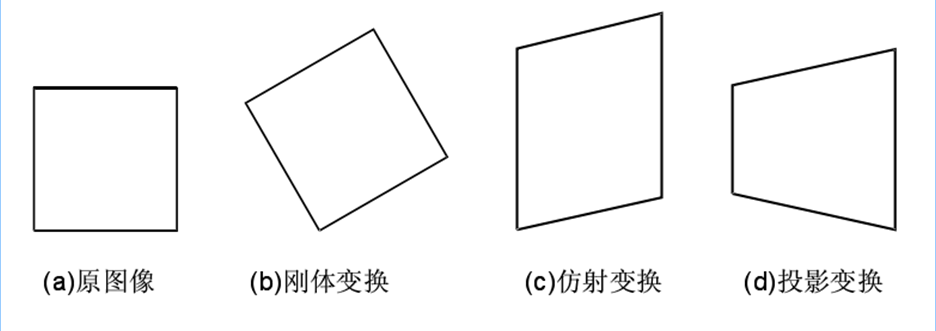(1) 刚体变换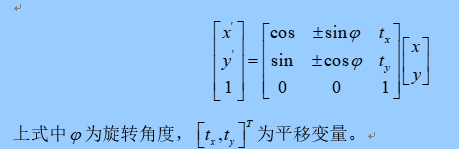(2) 仿射变换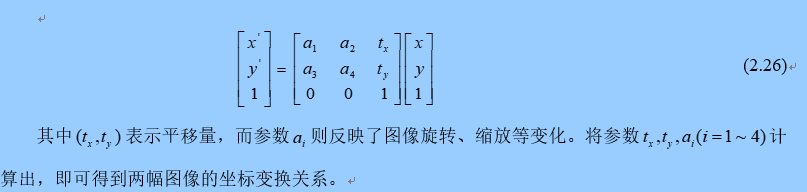(3) 投影变换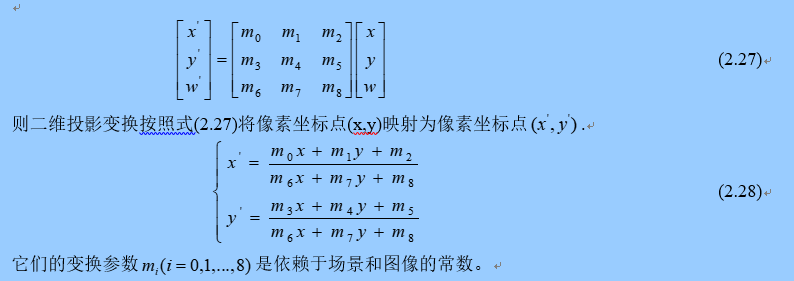(4) 非线性变换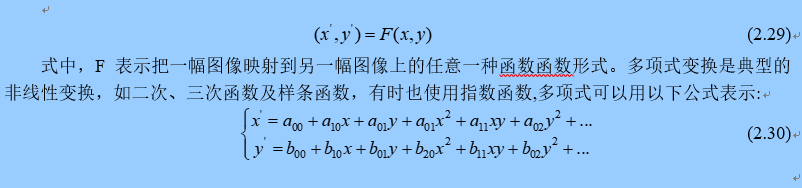## 图像的变换模型

2016-06-07 15:37:10 shenshen211 阅读数 1270
• ###### 基于计算机视觉的无人驾驶感知系统

AI技术落地，给你的履历增加一个厉害的实训项目！ 掌握无人驾驶技术开发的重要知识点，进行实战。

8637课时 0分钟 343人学习 王而川
免费试看

From:  遥感图像拼接算法研究1 刚体变换2  仿射变换3  投影变换

投影变换（Projective Transformation）是指变换过程中，图像中的直线只保持“平4  非线性变换## 【图像处理】图像几何变换基础（非弹性变换下如何计算旋转矩阵）

2016-05-23 14:37:51 lpsl1882 阅读数 3176
• ###### 基于计算机视觉的无人驾驶感知系统

AI技术落地，给你的履历增加一个厉害的实训项目！ 掌握无人驾驶技术开发的重要知识点，进行实战。

8637课时 0分钟 343人学习 王而川
免费试看

### 图像几何变换基础

我们实际获取的图像，常常因为各种原因，相对于原图有一定的几何形变，或者出于处理考虑，需要进行几何变换。二维图像坐标的几何变换写为：(x,y)=r(x,y)$(x^{'},y^{'})=r(x,y)$。根据图像几何变换的程度，可以将图像几何变换划分为如下几类：

• 刚体变换 如果一幅图像中的两点间的距离经变换到另一幅图像中后仍然保持不变，则这种变换称为刚体变换(Rigid Transform)。刚体变换仅局限于平移、旋转和反转(镜像)。
• 仿射变换 如果一幅图像中的直线经过后映射到另一幅图像上仍为直线，并且保持平行关系，则这种变换称为仿射变换(Affine Transform。仿射变换适应于平移、旋转、缩放和反转(镜像)情况。
• 投影变换 如果一幅图像中的直线经过后映射到另一幅图像上仍为直线，但平行关系基本不保持，则这种变换称为投影变换(Projective Transform )。
• 非线性变换 非线性变换又称为弯曲变换(Curved Transform)或者弹性变换，经过非线性变换，一幅图像上的直线映射到另一幅图像上不一定是直线，可能是曲线。

xkykwk=a11xmk+a12xm1kyk+...a1mymk+a1wk=a21xmk+a22xm1kyk+...a2mymk+a2wk=a31xmk+a32xm1kyk+...a3mymk+a3wk,n=1,2,3...(1)

xkykwk=a1xk+a2yk+a3wk=a4xk+a5yk+a6wk=a7xk+a8yk+a9wk,k=1,2,3...n(2)

same as x1x2x3...xny1y2y3ynw1w2w3wn=a1a4a7a2a5a8a3a6a9x1x2x3...xny1y2y3ynw1w2w3wnB=AX(3)

A称为空间变换矩阵。其中[a1a4a2a5]$\begin{bmatrix} a_1 & a_2 \\ a_4 & a_5 \end{bmatrix}$控制旋转、缩放和斜切，[a7a8]$\begin{bmatrix} a_7 & a_8 \end{bmatrix}$控制平移，[a3a6]$\begin{bmatrix} a_3 & a_6 \end{bmatrix}$控制透视变换；w是归一化因子，要求wk$w_k^{'}$wk$w_k$都是1，在特殊情况下为了便于分析可以不为1。三维图像空间变换同理，扩展出z变量，组成一个4×4$4\times4$的变换矩阵即可。
如果我们知道需要做何种变换，直接在单位空间变化矩阵上修改相应系数即可；如果我们不知道图像相比原图像做了什么变换，那么就需要设法获取图像的变换信息。常见的方法是手工标记前后两幅图像中的对应特征点，根据特征点的变换来计算得到变换的矩阵。
我这里的计算限制于透视变换，或者非弹性变换。当我们获取足够的变换特征点信息，就可以计算变换矩阵，方法是求解(2)式中的系数矩阵A。这里单独看方程式很像求解多元一次方程组，但是多元一次方程组求解的是x,y而不是系数；求解系数矩阵的一个著名方法，前面在【机器学习】线性回归小结提到，即最小二乘法。
假设我要变换一个256x256的图像，目标图像的四个边角坐标为(0,0),(180,75),(255,255),(75,180)。由于图像通常是方形的，因此四个边角点可以作为变换特征点。在《数字图像处理》书中，对四边形的空间变换使用了双线性方程来描述，这里我们直接使用空间变换矩阵，写成：
0180255750752551801111=a1a4a7a2a5a8a3a6a902552550002552551111

A0.7060.29400.2940.7060001## 图像变换——计算机视觉图像处理

2014-02-22 20:54:38 lxy201700 阅读数 2383
• ###### 基于计算机视觉的无人驾驶感知系统

AI技术落地，给你的履历增加一个厉害的实训项目！ 掌握无人驾驶技术开发的重要知识点，进行实战。

8637课时 0分钟 343人学习 王而川
免费试看

仿射变换的性质:平面上任意两条直线，经仿射变换后，仍然保持平行。

此类变换可以用一个3×3的矩阵来表示，其最后一行为(0, 0, 1)。该变换矩阵将原坐标(x, y)变换为新坐标(x', y')，这里原坐标和新坐标皆视为最末一行为(1)的三维列向量，原列向量左乘变换矩阵得到新的列向量：

[x']     [a00 a01       a02] [x]      [a00*x+a01*y+a02]

[y'] = [a10 a11 a12] [y] = [a10*x+a11*y+a12]

[1 ]         [0    0 1]       [ 1           ]

几种典型的仿射变换：

[ 1 0 tx   ]

[ 0 1 ty   ]

[ 0 0 1 ]

[ sx 0 0 ]

[ 0 sy 0 ]

[ 0 0 1 ]

[ 1 shx 0 ]

[   shy 1 0 ]

[ 0     0 1 ]

[ 1    0 0 ][ 1 shx 0 ]

[   shy 1 0 ][ 0     1     0 ]

[ 0    0 1 ][ 0 0     1 ]

“剪切变换”又称“错切变换”，指的是类似于四边形不稳定性那种性质，街边小商店那种铁拉门都见过吧？想象一下上面铁条构成的菱形拉动的过程，那就是“错切”的过程。

[ cos(theta) -sin(theta) 0 ]

[ sin(theta)     cos(theta) 0 ]

[    0          0          1 ]

[ cos(theta) -sin(theta) x-x*cos+y*sin]

[ sin(theta)     cos(theta) y-x*sin-y*cos ]

[    0               0           1       ]

[1   0   -x] [cos(theta)   -sin(theta)   0] [1   0   x]

[0   1   -y] [sin(theta) cos(theta)   0] [0   1   y]

[0   0   1 ] [     0         0        1 ] [0   0   1]

opencv中的实现函数：

void cvWarpAffine( const CvArr* src, CvArr* dst, const CvMat* map_matrix,

int flags=CV_INTER_LINEAR+CV_WARP_FILL_OUTLIERS,

CvScalar fillval=cvScalarAll(0) );

CvMat* cvGetAffineTransform( const CvPoint2D32f* src, const CvPoint2D32f* dst,

CvMat* map_matrix ); //由三个不共线的点得到，这样才具有唯一性

CvMat* cv2DRotationMatrix( CvPoint2D32f center, double angle, double scale,

CvMat* map_matrix ); //由旋转中心，旋转角度和各向通行因子，矩阵如下：

[ a b (1-a)*center.x-b*center.y ]

[-b a b*center.x-(1-a)*center.y ] 其中：a=scale*cos(angle), b=scale*sin(angle)

void cvGetQuadrangleSubPix( const CvArr* src, CvArr* dst, const CvMat* map_matrix );

#include "cv.h"
#include "highgui.h"
#include "math.h"
int main(int argc, char* argv[])
{
IplImage *src = 0;
IplImage *dst = 0;
IplImage *dst1 = 0;

/* the first command line parameter must be image file name */
int delta = 1;
int angle = 0;
int opt = 0;
// 1： 旋转加缩放
// 0: 仅仅旋转
double factor;

dst = cvCloneImage (src);
dst1 = cvCloneImage (src);
cvNamedWindow ("src", 1);
cvShowImage ("src", src);

for (;;)
{
float m;
// Matrix m looks like:
//
// [ m0 m1 m2 ] ===> [ A11 A12   b1 ]
// [ m3 m4 m5 ]       [ A21 A22   b2 ]
//
CvMat M = cvMat (2, 3, CV_32F, m);
int w = src->width;
int h = src->height;
if (opt)           // 旋转加缩放
factor = (cos (angle * CV_PI / 180.) + 1.0) * 2;
else                // 仅仅旋转
factor = 1;
m = (float) (factor * cos (-angle * 2 * CV_PI / 180.));
m = (float) (factor * sin (-angle * 2 * CV_PI / 180.));
m = -m;
m = m;
// 将旋转中心移至图像中间
m = w * 0.5f;
m = h * 0.5f;
// dst(x,y) = A * src(x,y) + b
cvZero (dst);
cvNamedWindow ("dst", 1);
cvShowImage ("dst", dst);
if (cvWaitKey (1) == 27)       //ESC
break;
angle = (int) (angle + delta) % 360;
}                   // for-loop

return 0;
}  

[ m0 m1 m2 ]

[ m3 m4 m5 ]

m0 = factor * cos (angle);

m1 = factor * sin (angle);

m3 = - factor * sin (angle);

m4 = factor * cos (angle);

m2 = x * (1- factor * cos (angle)) – y * factor * sin (angle); //x * (1-m0) – y * m1

m5 = y * (1- factor * cos (angle)) + x * factor * sin (angle); //y * (1-m0) + x* m1

[ m0 m1 m2 ]

[ m3 m4 m5 ]

m0 = factor * cos (angle); //同前

m1 = factor * sin (angle); //同前

m3 = - factor * sin (angle); //同前

m4 = factor * cos (angle); //同前

m2 = x;

m5 = y;

opencv中的实现函数：

void cvWarpPerspective( const CvArr* src, CvArr* dst, const CvMat* map_matrix, int flags=CV_INTER_LINEAR+CV_WARP_FILL_OUTLIERS, CvScalar fillval=cvScalarAll(0));

CvMat* cvGetPerspectiveTransform( const CvPoint2D32f* src, const CvPoint2D32f* dst, CvMat* map_matrix ); //用四边形的四个点得到





## 关于图像变换的总结（仿射变换，刚体变换等）

2019-07-10 21:14:13 qq_33590958 阅读数 705
• ###### 基于计算机视觉的无人驾驶感知系统

AI技术落地，给你的履历增加一个厉害的实训项目！ 掌握无人驾驶技术开发的重要知识点，进行实战。

8637课时 0分钟 343人学习 王而川
免费试看

:刚性变换、仿射变换、透视变换和非线形变换等。如下图所示：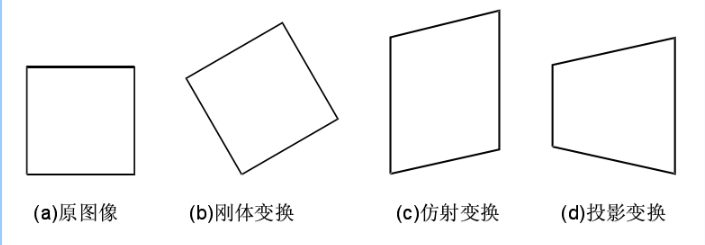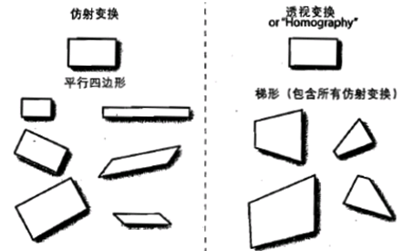(1) 刚体变换

(2)仿射变换

(3) 投影变换

Affine Transformation是一种二维坐标到二维坐标之间的线性变换，保持二维图形的“平直性”（译注：straightness，即变换后直线还是直线不会打弯，圆弧还是圆弧）和“平行性”（译注：parallelness，其实是指保二维图形间的相对位置关系不变，平行线还是平行线）。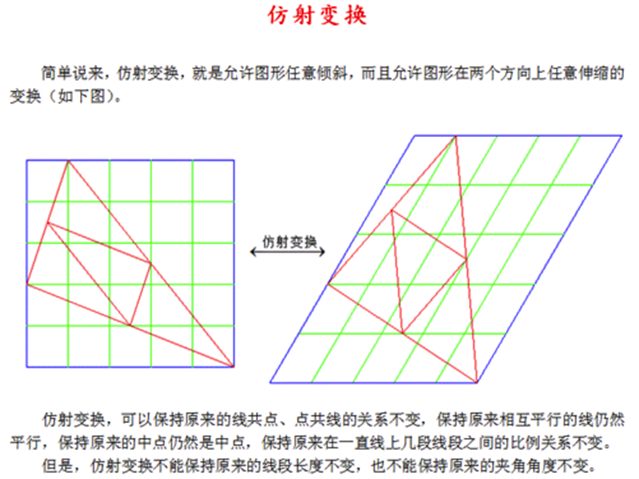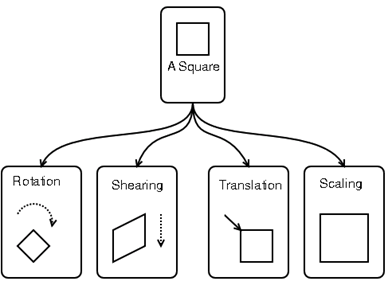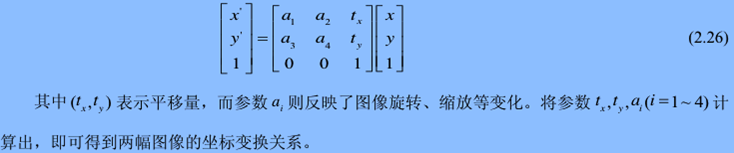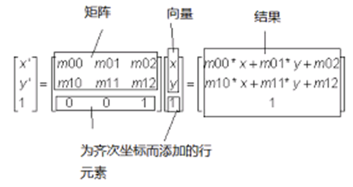X = x/w
Y = y/w